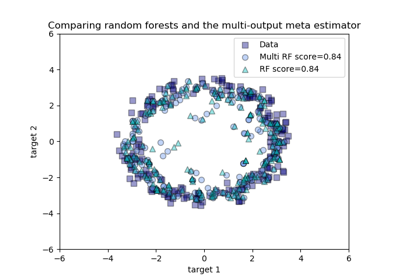# sklearn.multioutput.MultiOutputRegressor¶

class sklearn.multioutput.MultiOutputRegressor(estimator, n_jobs=None)[source]

Multi target regression

This strategy consists of fitting one regressor per target. This is a simple strategy for extending regressors that do not natively support multi-target regression.

Parameters: estimator : estimator object An estimator object implementing fit and predict. n_jobs : int or None, optional (default=None) The number of jobs to run in parallel for fit. None means 1 unless in a joblib.parallel_backend context. -1 means using all processors. See Glossary for more details. When individual estimators are fast to train or predict using n_jobs>1 can result in slower performance due to the overhead of spawning processes. estimators_ : list of n_output estimators Estimators used for predictions.

Methods

 fit(self, X, y[, sample_weight]) Fit the model to data. get_params(self[, deep]) Get parameters for this estimator. partial_fit(self, X, y[, sample_weight]) Incrementally fit the model to data. predict(self, X) Predict multi-output variable using a model trained for each target variable. score(self, X, y[, sample_weight]) Returns the coefficient of determination R^2 of the prediction. set_params(self, \*\*params) Set the parameters of this estimator.
__init__(self, estimator, n_jobs=None)[source]
fit(self, X, y, sample_weight=None)[source]

Fit the model to data. Fit a separate model for each output variable.

Parameters: X : (sparse) array-like, shape (n_samples, n_features) Data. y : (sparse) array-like, shape (n_samples, n_outputs) Multi-output targets. An indicator matrix turns on multilabel estimation. sample_weight : array-like, shape = (n_samples) or None Sample weights. If None, then samples are equally weighted. Only supported if the underlying regressor supports sample weights. self : object
get_params(self, deep=True)[source]

Get parameters for this estimator.

Parameters: deep : boolean, optional If True, will return the parameters for this estimator and contained subobjects that are estimators. params : mapping of string to any Parameter names mapped to their values.
partial_fit(self, X, y, sample_weight=None)[source]

Incrementally fit the model to data. Fit a separate model for each output variable.

Parameters: X : (sparse) array-like, shape (n_samples, n_features) Data. y : (sparse) array-like, shape (n_samples, n_outputs) Multi-output targets. sample_weight : array-like, shape = (n_samples) or None Sample weights. If None, then samples are equally weighted. Only supported if the underlying regressor supports sample weights. self : object
predict(self, X)[source]
Predict multi-output variable using a model
trained for each target variable.
Parameters: X : (sparse) array-like, shape (n_samples, n_features) Data. y : (sparse) array-like, shape (n_samples, n_outputs) Multi-output targets predicted across multiple predictors. Note: Separate models are generated for each predictor.
score(self, X, y, sample_weight=None)[source]

Returns the coefficient of determination R^2 of the prediction.

The coefficient R^2 is defined as (1 - u/v), where u is the residual sum of squares ((y_true - y_pred) ** 2).sum() and v is the regression sum of squares ((y_true - y_true.mean()) ** 2).sum(). Best possible score is 1.0 and it can be negative (because the model can be arbitrarily worse). A constant model that always predicts the expected value of y, disregarding the input features, would get a R^2 score of 0.0.

Parameters: X : array-like, shape (n_samples, n_features) Test samples. y : array-like, shape (n_samples) or (n_samples, n_outputs) True values for X. sample_weight : array-like, shape [n_samples], optional Sample weights. score : float R^2 of self.predict(X) wrt. y.

Notes

R^2 is calculated by weighting all the targets equally using multioutput='uniform_average'.

set_params(self, **params)[source]

Set the parameters of this estimator.

The method works on simple estimators as well as on nested objects (such as pipelines). The latter have parameters of the form <component>__<parameter> so that it’s possible to update each component of a nested object.

Returns: self

## Examples using sklearn.multioutput.MultiOutputRegressor¶Comparing random forests and the multi-output meta estimator